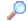Search
 Module: [All]advapi32avifil32aygshellcardscfgmgr32comctl32comdlg32Constantscoredllcreduicrypt32dbghelpdbghlpdbghlp32Delegatesdhcpsapidifxapidmcl40dnsapidtldwmapiEnumsfaultrepfbwflibfltlibfwpuclntgdi32gdiplusgetunameglossaryglu32glut32gsapihhctrlhidhlinkhttpapiicmpimm32Interfacesipaqutiliphlpapiipropirpropskernel32mapi32MinCoremiscmprmqrtmscorsnmsdeltamsdrmmsimsportsmsvcrtncryptnetapi32ntdllntdsapiodbc32odbccp32ole32oleaccoleaut32opengl32pdhpowrprofprintuipropsyspsapipstorecqueryquickusbrapirasapi32rpcrt4scarddlgsecur32setupapishell32shlwapiStructurestwain_32unicowsurlmonuser32userenvuxthemeversionwerwevtapiwinfaxwinhttpwininetwinmmwinscardwinspoolwintrustwinusbwlanapiws2_32wtsapi32xolehlpxpsprint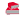Directory

Desktop Functions:

Smart Device Functions: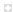Glossary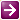Show Recent ChangesSubscribe (RSS)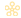Misc. Pages
SendInput (user32)

Summary

The SendInput function synthesizes keystrokes, mouse motions, and button clicks to the currently active window

#### C# Signature:

[DllImport("user32.dll", SetLastError=true)]
static extern uint SendInput(uint nInputs, INPUT [] pInputs, int cbSize);

#### VB.NET Signature:

<DllImport("user32.dll", SetLastError=true)> _
Private Shared Function SendInput(ByVal nInputs As Integer, ByRef pInputs As INPUT, byval cbSize As Integer) As Integer
End Function

Private Declare Function SendInput Lib "user32.dll" (ByVal cInputs As Integer, ByRef pInputs As INPUT, ByVal cbSize As Integer) As Integer

#### C# User-Defined Types:

struct MOUSEINPUT
{
public int dx;
public int dy;
public uint mouseData;
public uint dwFlags;
public uint time;
public IntPtr dwExtraInfo;
}

struct KEYBDINPUT
{
public ushort wVk;
public ushort wScan;
public uint dwFlags;
public uint time;
public IntPtr dwExtraInfo;
}

struct HARDWAREINPUT
{
public uint uMsg;
public ushort wParamL;
public ushort wParamH;
}

[StructLayout(LayoutKind.Explicit)]
struct MOUSEKEYBDHARDWAREINPUT
{
[FieldOffset(0)]
public MOUSEINPUT mi;

[FieldOffset(0)]
public KEYBDINPUT ki;

[FieldOffset(0)]
public HARDWAREINPUT hi;
}

struct INPUT
{
public int type;
public MOUSEKEYBDHARDWAREINPUT mkhi;
}

[DllImport("user32.dll")]
static extern IntPtr GetMessageExtraInfo();

#### VB.NET User-Defined Types:

Private Structure INPUT
Dim dwType As Integer
Dim mkhi as MOUSEKEYBDHARDWAREINPUT
End Structure

Private Structure KEYBDINPUT
Public wVk As Short
Public wScan As Short
Public dwFlags As Integer
Public time As Integer
Public dwExtraInfo As IntPtr
End Structure

Private Structure HARDWAREINPUT
Public uMsg As Integer
Public wParamL As Short
Public wParamH As Short
End Structure

<StructLayout(LayoutKind.Explicit)> _
Private Structure MOUSEKEYBDHARDWAREINPUT
<FieldOffset(0)> Public mi As MOUSEINPUT
<FieldOffset(0)> Public ki As KEYBDINPUT
<FieldOffset(0)> Public hi As HARDWAREINPUT
End Structure

Private Structure MOUSEINPUT
Public dx As Integer
Public dy As Integer
Public mouseData As Integer
Public dwFlags As Integer
Public time As Integer
Public dwExtraInfo As IntPtr
End Structure

#### Notes:

You'll need some constants to use this function. Here they are.

const int INPUT_MOUSE          = 0;
const int INPUT_KEYBOARD       = 1;
const int INPUT_HARDWARE       = 2;
const uint KEYEVENTF_EXTENDEDKEY   = 0x0001;
const uint KEYEVENTF_KEYUP     = 0x0002;
const uint KEYEVENTF_UNICODE       = 0x0004;
const uint KEYEVENTF_SCANCODE      = 0x0008;
const uint XBUTTON1        = 0x0001;
const uint XBUTTON2        = 0x0002;
const uint MOUSEEVENTF_MOVE    = 0x0001;
const uint MOUSEEVENTF_LEFTDOWN    = 0x0002;
const uint MOUSEEVENTF_LEFTUP      = 0x0004;
const uint MOUSEEVENTF_RIGHTDOWN   = 0x0008;
const uint MOUSEEVENTF_RIGHTUP     = 0x0010;
const uint MOUSEEVENTF_MIDDLEDOWN  = 0x0020;
const uint MOUSEEVENTF_MIDDLEUP    = 0x0040;
const uint MOUSEEVENTF_XDOWN       = 0x0080;
const uint MOUSEEVENTF_XUP     = 0x0100;
const uint MOUSEEVENTF_WHEEL       = 0x0800;
const uint MOUSEEVENTF_VIRTUALDESK = 0x4000;
const uint MOUSEEVENTF_ABSOLUTE    = 0x8000;

Here are the VB.Net constants:

Const INPUT_MOUSE As UInt32 = 0
Const INPUT_KEYBOARD As Integer = 1
Const INPUT_HARDWARE As Integer = 2
Const KEYEVENTF_EXTENDEDKEY As UInt32 = &H1
Const KEYEVENTF_KEYUP As UInt32 = &H2
Const KEYEVENTF_UNICODE As UInt32 = &H4
Const KEYEVENTF_SCANCODE As UInt32 = &H8
Const XBUTTON1 As UInt32 = &H1
Const XBUTTON2 As UInt32 = &H2
Const MOUSEEVENTF_MOVE As UInt32 = &H1
Const MOUSEEVENTF_LEFTDOWN As UInt32 = &H2
Const MOUSEEVENTF_LEFTUP As UInt32 = &H4
Const MOUSEEVENTF_RIGHTDOWN As UInt32 = &H8
Const MOUSEEVENTF_RIGHTUP As UInt32 = &H10
Const MOUSEEVENTF_MIDDLEDOWN As UInt32 = &H20
Const MOUSEEVENTF_MIDDLEUP As UInt32 = &H40
Const MOUSEEVENTF_XDOWN As UInt32 = &H80
Const MOUSEEVENTF_XUP As UInt32 = &H100
Const MOUSEEVENTF_WHEEL As UInt32 = &H800
Const MOUSEEVENTF_VIRTUALDESK As UInt32 = &H4000
Const MOUSEEVENTF_ABSOLUTE As UInt32 = &H8000

### Virtual-Key-Codes constants [C#]

// enum : VK

public enum VK : ushort
{
/*
* Virtual Keys, Standard Set */         VK_LBUTTON = 0x01,
VK_RBUTTON = 0x02,
VK_CANCEL =  0x03,
VK_MBUTTON = 0x04,    /* NOT contiguous with L & RBUTTON */

VK_XBUTTON1 = 0x05,    /* NOT contiguous with L & RBUTTON */
VK_XBUTTON2 = 0x06,    /* NOT contiguous with L & RBUTTON */

/*
* 0x07 : unassigned */
VK_BACK =    0x08,
VK_TAB =     0x09,

/*
* 0x0A - 0x0B : reserved */
VK_CLEAR =   0x0C,
VK_RETURN =  0x0D,

VK_SHIFT =   0x10,
VK_CONTROL = 0x11,
VK_MENU =    0x12,
VK_PAUSE =   0x13,
VK_CAPITAL = 0x14,

VK_KANA =    0x15,
VK_HANGEUL = 0x15,  /* old name - should be here for compatibility */
VK_HANGUL =  0x15,
VK_JUNJA =   0x17,
VK_FINAL =   0x18,
VK_HANJA =   0x19,
VK_KANJI =   0x19,

VK_ESCAPE =  0x1B,

VK_CONVERT =    0x1C,
VK_NONCONVERT = 0x1D,
VK_ACCEPT =     0x1E,
VK_MODECHANGE = 0x1F,

VK_SPACE =   0x20,
VK_PRIOR =   0x21,
VK_NEXT =    0x22,
VK_END =     0x23,
VK_HOME =    0x24,
VK_LEFT =    0x25,
VK_UP =      0x26,
VK_RIGHT =   0x27,
VK_DOWN =    0x28,
VK_SELECT =  0x29,
VK_PRINT =   0x2A,
VK_EXECUTE = 0x2B,
VK_SNAPSHOT = 0x2C,
VK_INSERT =  0x2D,
VK_DELETE =  0x2E,
VK_HELP =    0x2F,

/*
* VK_0 - VK_9 are the same as ASCII '0' - '9' (0x30 - 0x39) * 0x40 : unassigned * VK_A - VK_Z are the same as ASCII 'A' - 'Z' (0x41 - 0x5A) */
VK_LWIN =    0x5B,
VK_RWIN =    0x5C,
VK_APPS =    0x5D,

/*
* 0x5E : reserved */
VK_SLEEP =   0x5F,

VK_NUMPAD0 = 0x60,
VK_NUMPAD1 = 0x61,
VK_NUMPAD2 = 0x62,
VK_NUMPAD3 = 0x63,
VK_NUMPAD4 = 0x64,
VK_NUMPAD5 = 0x65,
VK_NUMPAD6 = 0x66,
VK_NUMPAD7 = 0x67,
VK_NUMPAD8 = 0x68,
VK_NUMPAD9 = 0x69,
VK_MULTIPLY = 0x6A,
VK_ADD = 0x6B,
VK_SEPARATOR = 0x6C,
VK_SUBTRACT  = 0x6D,
VK_DECIMAL = 0x6E,
VK_DIVIDE =  0x6F,
VK_F1 =      0x70,
VK_F2 =      0x71,
VK_F3 =      0x72,
VK_F4 =      0x73,
VK_F5 =      0x74,
VK_F6 =      0x75,
VK_F7 =      0x76,
VK_F8 =      0x77,
VK_F9 =      0x78,
VK_F10 =     0x79,
VK_F11 =     0x7A,
VK_F12 =     0x7B,
VK_F13 =     0x7C,
VK_F14 =     0x7D,
VK_F15 =     0x7E,
VK_F16 =     0x7F,
VK_F17 =     0x80,
VK_F18 =     0x81,
VK_F19 =     0x82,
VK_F20 =     0x83,
VK_F21 =     0x84,
VK_F22 =     0x85,
VK_F23 =     0x86,
VK_F24 =     0x87,

/*
* 0x88 - 0x8F : unassigned */
VK_NUMLOCK = 0x90,
VK_SCROLL =  0x91,

/*
* VK_L* & VK_R* - left and right Alt, Ctrl and Shift virtual keys. * Used only as parameters to GetAsyncKeyState() and GetKeyState(). * No other API or message will distinguish left and right keys in this way. */         VK_LSHIFT   = 0xA0,
VK_RSHIFT   = 0xA1,
VK_LCONTROL = 0xA2,
VK_RCONTROL = 0xA3,
VK_LMENU    = 0xA4,
VK_RMENU    = 0xA5,

VK_BROWSER_BACK    = 0xA6,
VK_BROWSER_FORWARD = 0xA7,
VK_BROWSER_REFRESH = 0xA8,
VK_BROWSER_STOP    = 0xA9,
VK_BROWSER_SEARCH    = 0xAA,
VK_BROWSER_FAVORITES = 0xAB,
VK_BROWSER_HOME    = 0xAC,

VK_VOLUME_MUTE =  0xAD,
VK_VOLUME_DOWN =  0xAE,
VK_VOLUME_UP =    0xAF,
VK_MEDIA_NEXT_TRACK = 0xB0,
VK_MEDIA_PREV_TRACK = 0xB1,
VK_MEDIA_STOP =   0xB2,
VK_MEDIA_PLAY_PAUSE = 0xB3,
VK_LAUNCH_MAIL =  0xB4,
VK_LAUNCH_MEDIA_SELECT = 0xB5,
VK_LAUNCH_APP1 =  0xB6,
VK_LAUNCH_APP2 =  0xB7,

/*
* 0xB8 - 0xB9 : reserved */
VK_OEM_1      = 0xBA,   // ';:' for US
VK_OEM_PLUS   = 0xBB,   // '+' any country
VK_OEM_COMMA  = 0xBC,   // ',' any country
VK_OEM_MINUS  = 0xBD,   // '-' any country
VK_OEM_PERIOD = 0xBE,   // '.' any country
VK_OEM_2      = 0xBF,   // '/?' for US
VK_OEM_3      = 0xC0,   // '`~' for US

/*
* 0xC1 - 0xD7 : reserved */
/*
* 0xD8 - 0xDA : unassigned */
VK_OEM_4 =   0xDB,  //  '[{' for US
VK_OEM_5 =   0xDC,  //  '\|' for US
VK_OEM_6 =   0xDD,  //  ']}' for US
VK_OEM_7 =   0xDE,  //  ''"' for US
VK_OEM_8 =   0xDF

/*
* 0xE0 : reserved */

}

#### Tips & Tricks:

Big GOTCHA: It is very unclear from Microsoft's documentation, but if you use a VK_ code, and that code represents a key that is an "Extended" key, you can get some strange responses. Things won't work for odd reasons. But, a line like:

if ((x >= 33 && x <= 46) || (x >= 91 && x <= 93)) ki.dwFlags += KEYEVENTF_EXTENDEDKEY;

will fix this. The affected keys are identifiable by E0 in the scan code. This is true even though scan codes and VK_ enumeration codes are different! It is the key that makes the difference. You have to declare it "extended" as in the code line above.

Another GOTCHA: Characters appear in text boxes just fine. BUT, if you want to send control codes such as <ctl>C, the system converts the unicode character to a packet and it will not work. In this case, you have to use "real" scan codes with appropriate modifier keys.

windows CE universal core virtual key code compact chart http://msdn2.microsoft.com/en-us/library/aa926323.aspx

Use FindWindow and SetFocus to direct input to the desired window.

Note
Sleep may be required to assure Window is ready for input:

RUN('NOTEPAD.EXE')
Sleep(2000,0)
SetFocus(FindWindow('Untitled - Notepad'))

#### Sample Code: WARNING: The C# code will not compile due to numerous errors.

// It might be better to write this code as a single call to SendInput passing
// all the key downs and keys ups in one array.  If you do this, SendInput
// guarantees that no other keys get inbetween the whole sequence.  As is stands,
// a "real" key transition could in theory sneak in the middle - and if it did
// it would inherit the states of shift, control, etc this program had set up.

class Test
{
public enum VK : ushort
{
SHIFT        = 0x10,
CONTROL          = 0x11,
MENU         = 0x12,
ESCAPE           = 0x1B,
BACK         = 0x08,
TAB          = 0x09,
RETURN           = 0x0D,
PRIOR        = 0x21,
NEXT         = 0x22,
END          = 0x23,
HOME         = 0x24,
LEFT         = 0x25,
UP           = 0x26,
RIGHT        = 0x27,
DOWN         = 0x28,
SELECT           = 0x29,
PRINT        = 0x2A,
EXECUTE          = 0x2B,
SNAPSHOT         = 0x2C,
INSERT           = 0x2D,
DELETE           = 0x2E,
HELP         = 0x2F,
NUMPAD0          = 0x60,
NUMPAD1          = 0x61,
NUMPAD2          = 0x62,
NUMPAD3          = 0x63,
NUMPAD4          = 0x64,
NUMPAD5          = 0x65,
NUMPAD6          = 0x66,
NUMPAD7          = 0x67,
NUMPAD8          = 0x68,
NUMPAD9          = 0x69,
MULTIPLY         = 0x6A,
ADD          = 0x6B,
SEPARATOR        = 0x6C,
SUBTRACT         = 0x6D,
DECIMAL          = 0x6E,
DIVIDE           = 0x6F,
F1           = 0x70,
F2           = 0x71,
F3           = 0x72,
F4           = 0x73,
F5           = 0x74,
F6           = 0x75,
F7           = 0x76,
F8           = 0x77,
F9           = 0x78,
F10          = 0x79,
F11          = 0x7A,
F12          = 0x7B,
OEM_1        = 0xBA,   // ',:' for US
OEM_PLUS         = 0xBB,   // '+' any country
OEM_COMMA        = 0xBC,   // ',' any country
OEM_MINUS        = 0xBD,   // '-' any country
OEM_PERIOD       = 0xBE,   // '.' any country
OEM_2        = 0xBF,   // '/?' for US
OEM_3        = 0xC0,   // '`~' for US
MEDIA_NEXT_TRACK     = 0xB0,
MEDIA_PREV_TRACK     = 0xB1,
MEDIA_STOP           = 0xB2,
MEDIA_PLAY_PAUSE     = 0xB3,
LWIN       =0x5B,
RWIN       =0x5C
}

public struct KEYBDINPUT
{
public ushort wVk;
public ushort wScan;
public uint dwFlags;
public long time;
public uint dwExtraInfo;
};
[StructLayout(LayoutKind.Explicit,Size=28)]
public struct INPUT
{
public uint type;
public KEYBDINPUT ki;
};

void Test()
{
uint intReturn;
INPUT structInput;
structInput = new INPUT();
structInput.type = Win32Consts.INPUT_KEYBOARD;

// Key down shift, ctrl, and/or alt
structInput.mkhi.ki.wScan = 0;
structInput.mkhi.ki.time = 0;
structInput.mkhi.ki.dwFlags = 0;
if (Shift)
{
structInput.mkhi.ki.wVk = (ushort)VK.SHIFT;
intReturn = Win32.SendInput(1, ref structInput, (UInt32)sizeof(INPUT));
}
if (Ctrl)
{
structInput.mkhi.ki.wVk = (ushort)VK.CONTROL;
intReturn = Win32.SendInput(1, ref structInput, (UInt32)sizeof(INPUT));
}
if (Alt)
{
structInput.mkhi.ki.wVk = (ushort)VK.MENU;
intReturn = Win32.SendInput(1, ref structInput, (UInt32)sizeof(INPUT));
}
if (Win)
{
structInput.mkhi.ki.wVk = (ushort)VK.LWIN;
intReturn = Win32.SendInput(1, ref structInput, (UInt32)sizeof(INPUT));
}
// Key down the actual key-code
structInput.mkhi.ki.wVk = vk;
intReturn = Win32.SendInput(1, ref structInput, (UInt32)sizeof(INPUT));
// Key up the actual key-code
structInput.mkhi.ki.dwFlags = Win32Consts.KEYEVENTF_KEYUP;
intReturn = Win32.SendInput(1, ref structInput, (UInt32)sizeof(INPUT));
// Key up shift, ctrl, and/or alt
if (Shift)
{
structInput.mkhi.ki.wVk = (ushort)VK.SHIFT;
intReturn = Win32.SendInput(1, ref structInput, (UInt32)sizeof(INPUT));
}
if (Ctrl)
{
structInput.mkhi.ki.wVk = (ushort)VK.CONTROL;
intReturn = Win32.SendInput(1, ref structInput, (UInt32)sizeof(INPUT));
}
if (Alt)
{
structInput.mkhi.ki.wVk = (ushort)VK.MENU;
intReturn = Win32.SendInput(1, ref structInput, (UInt32)sizeof(INPUT));
}
if (Win)
{
structInput.mkhi.ki.wVk = (ushort)VK.LWIN;
intReturn = Win32.SendInput(1, ref structInput, (UInt32)sizeof(INPUT));
}
}
}

#### VB.NET Sample Code:

Imports System.Runtime.InteropServices

Public Class Form1

Private tb As New TextBox
Private WithEvents but1 As New Button

Sub New()
InitializeComponent()
tb = New TextBox : tb.Location = New Point(10, 10)
but1 = New Button : but1.Location = New Point(10, 40)
but1.Text = "example"
Me.Controls.Add(but1) : Me.Controls.Add(tb)
End Sub

Private Sub DoMouse(ByVal flags As NativeMethods.MOUSEEVENTF, ByVal newPoint As Point)
Dim input As New NativeMethods.INPUT
Dim mi As New NativeMethods.MOUSEINPUT
input.dwType = NativeMethods.InputType.Mouse
input.mkhi.mi = mi
input.mkhi.mi.dwExtraInfo = IntPtr.Zero
' mouse co-ords: top left is (0,0), bottom right is (65535, 65535)
' convert screen co-ord to mouse co-ords...
input.mkhi.mi.dx = newPoint.X * (65535 / Screen.PrimaryScreen.Bounds.Width)
input.mkhi.mi.dy = newPoint.Y * (65535 / Screen.PrimaryScreen.Bounds.Height)
input.mkhi.mi.time = 0
input.mkhi.mi.mouseData = 0  ' can be used for WHEEL event see msdn
input.mkhi.mi.dwFlags = flags
Dim cbSize As Integer = Marshal.SizeOf(GetType(NativeMethods.INPUT))
Dim result As Integer = NativeMethods.SendInput(1, input, cbSize)
If result = 0 Then Debug.WriteLine(Marshal.GetLastWin32Error)
End Sub

Private Sub DoKeyBoard(ByVal flags As NativeMethods.KEYEVENTF, ByVal key As Keys)
Dim input As New NativeMethods.INPUT
Dim ki As New NativeMethods.KEYBDINPUT
input.dwType = NativeMethods.InputType.Keyboard
input.mkhi.ki = ki
input.mkhi.ki.wVk = Convert.ToInt16(key)
input.mkhi.ki.wScan = 0
input.mkhi.ki.time = 0
input.mkhi.ki.dwFlags = flags
input.mkhi.ki.dwExtraInfo = IntPtr.Zero
Dim cbSize As Integer = Marshal.SizeOf(GetType(NativeMethods.INPUT))
Dim result As Integer = NativeMethods.SendInput(1, input, cbSize)
If result = 0 Then Debug.WriteLine(Marshal.GetLastWin32Error)
End Sub

Private Sub but1_Click(ByVal sender As Object, ByVal e As System.EventArgs) Handles but1.Click
'move to the textbox, click it to focus, keydown some chars...
' get textbox location in screen co-ords
Dim tbLocation As Point = Me.PointToScreen(Me.tb.Location)
' nudge it into the tb
tbLocation.X += 5
tbLocation.Y += 5
' move to the TB. it is a MOVE event, and we use ABSOLUTE co-ordinates
DoMouse(NativeMethods.MOUSEEVENTF.MOVE Or NativeMethods.MOUSEEVENTF.ABSOLUTE, tbLocation)
' click the TB
DoMouse(NativeMethods.MOUSEEVENTF.LEFTDOWN, New Point(0, 0))
' release the mouse
DoMouse(NativeMethods.MOUSEEVENTF.LEFTUP, New Point(0, 0))
' the key codes are virtual keycodes (see mdsn)
' I'm using a shortcut to save space - casting chars to ints
' if you want to change case, you need to send a shift key with the letter...
' sbq: I found the routine VkKeyScanA very helpful converting from
'      characters to virtual key codes.  Look it up in the MSDN.  I declared it
'      as shown below.  It returns the key code in the low byte and the additional
'      keys to be pressed while transmitting in the upper byte (e.g., shift, ctrl, alt):
'
'      Private Declare Function VkKeyScanA Lib "user32" Alias "VkKeyScanA" (ByVal cChar_Renamed As Byte) As Short
'
Dim message As String = "HELLO WORLD"
For Each c As Char In message
Dim key As UInteger = Convert.ToInt16(c)
DoKeyBoard(0, key)  ' key down is 0 - no flag...
DoKeyBoard(NativeMethods.KEYEVENTF.KEYUP, key)
Next
DoKeyBoard(0, Keys.Decimal) ' example using keys enum which I think matches the VKEY values
DoKeyBoard(NativeMethods.KEYEVENTF.KEYUP, Keys.Decimal)
End Sub

End Class

Public Class NativeMethods

<DllImport("user32.dll", SetLastError:=True)> _
Friend Shared Function SendInput(ByVal cInputs As Int32, ByRef pInputs As INPUT, ByVal cbSize As Int32) As Int32
End Function

<StructLayout(LayoutKind.Explicit, pack:=1, Size:=28)> _
Friend Structure INPUT
<FieldOffset(0)> Public dwType As InputType
<FieldOffset(4)> Public mi As MOUSEINPUT
<FieldOffset(4)> Public ki As KEYBDINPUT
<FieldOffset(4)> Public hi As HARDWAREINPUT
End Structure

<StructLayout(LayoutKind.Sequential, pack:=1)> _
Friend Structure MOUSEINPUT
Public dx As Int32
Public dy As Int32
Public mouseData As Int32
Public dwFlags As MOUSEEVENTF
Public time As Int32
Public dwExtraInfo As IntPtr
End Structure

<StructLayout(LayoutKind.Sequential, pack:=1)> _
Friend Structure KEYBDINPUT
Public wVk As Int16
Public wScan As Int16
Public dwFlags As KEYEVENTF
Public time As Int32
Public dwExtraInfo As IntPtr
End Structure

<StructLayout(LayoutKind.Sequential, pack:=1)> _
Friend Structure HARDWAREINPUT
Public uMsg As Int32
Public wParamL As Int16
Public wParamH As Int16
End Structure

Friend Enum InputType As Integer
Mouse = 0
Keyboard = 1
Hardware = 2
End Enum

<Flags()> _
Friend Enum MOUSEEVENTF As Integer
MOVE = &H1
LEFTDOWN = &H2
LEFTUP = &H4
RIGHTDOWN = &H8
RIGHTUP = &H10
MIDDLEDOWN = &H20
MIDDLEUP = &H40
XDOWN = &H80
XUP = &H100
VIRTUALDESK = &H400
WHEEL = &H800
ABSOLUTE = &H8000
End Enum

<Flags()> _
Friend Enum KEYEVENTF As Integer
EXTENDEDKEY = 1
KEYUP = 2
[UNICODE] = 4
SCANCODE = 8
End Enum
End Class

* the above code does not work as it's trying to convert key into int16. When you want to send a Control plus some key sequence it generates an exception at that. Control translates to 131072***

Please note that there is an unresolved issue using the SendKeys class in Windows Vista RC1 (Build 5536).

More specifically you may encounter a "Hook cannot be created." exception.

Note

Please also note that there is an unresolved issue using the SendKeys class if ie7 is the target app.

More specifically, extra characters may be added to the input stream.

I was able to send keystrokes (or half keystrokes, i.e. KeyDown - followed sometime later by a KeyUp - for control purposes), sending them one at a time. I was unable to get an array of inputs to be accepted. (The additional keys I was trying to add were Shift, Ctrl, and Alt - so maybe that has some bearing.)

Do you know one? Please contribute it!

I found that using SendKeys to send {TAB} works OK but +{TAB} (i.e. shift-tab) doesn't; IE7 executes a forward-tab in both cases. Using the above routines, the following successfully does a shift-tab in IE7:

DoKeyBoard(0, Keys.ShiftKey)
DoKeyBoard(0, Keys.Tab)
DoKeyBoard(NativeMethods.KEYEVENTF.KEYUP, Keys.Tab)
DoKeyBoard(NativeMethods.KEYEVENTF.KEYUP, Keys.ShiftKey)

Documentation
SendInput on MSDN

# Please edit this page!

Do you have...

• helpful tips or sample code to share for using this API in managed code?
• corrections to the existing content?
• variations of the signature you want to share?
• additional languages you want to include?

Select "Edit This Page" on the right hand toolbar and edit it! Or add new pages containing supporting types needed for this API (structures, delegates, and more).Access PInvoke.net directly from VS:Terms of UseFind ReferencesShow Printable Version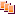Revisions Show changes